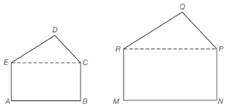Chapter 5.2, Problem 8E### Elementary Geometry for College St...

6th Edition
Daniel C. Alexander + 1 other
ISBN: 9781285195698

#### Solutions

Chapter
Section### Elementary Geometry for College St...

6th Edition
Daniel C. Alexander + 1 other
ISBN: 9781285195698
Textbook Problem
1 views

# Given that rectangle A B C E is similar to rectangle M N P R and that △ C D E ∼ △ P Q R , what can you conclude regarding pentagon A B C D E and pentagon M N P Q R ?To determine

To find: What can you conclude regarding pentagon ABCDE and pentagon MNPQR.

Explanation

Given:

The rectangle ABCE is similar to rectangle MNPR and ΔCDEΔPQR.

Definition used:

Two polygons are similar if and only if two conditions are satisfied:

1. All pairs of corresponding angles are congruent.

2. All pairs of corresponding sides are proportional.

Calculation:

We have ΔCDEΔPQR.

Thus the corresponding angles are congruent and the corresponding sides are proportional.

That is mD=mQ,mDEC=mQRP,mDCE=mQPR and

DCQP=DEQR=ECRP  ...(1)

Also we have rectangle ABCE is similar to rectangle MNPR.

Thus the corresponding angles are congruent and the corresponding sides are proportional.

That is, mCEA=mPRM,mECB=mRPN,mA=mM, mB=mN and

AEMR=ABMN=BCNP=ECRP

### Still sussing out bartleby?

Check out a sample textbook solution.

See a sample solution

#### The Solution to Your Study Problems

Bartleby provides explanations to thousands of textbook problems written by our experts, many with advanced degrees!

Get Started

#### Fill in the blanks. 8. a. The general form of an equation of a line is_______.

Applied Calculus for the Managerial, Life, and Social Sciences: A Brief Approach

#### The graph of x = cos t, y = sin2 t is:

Study Guide for Stewart's Multivariable Calculus, 8th

#### The graph of x = cos t, y = sin2 t is:

Study Guide for Stewart's Single Variable Calculus: Early Transcendentals, 8th